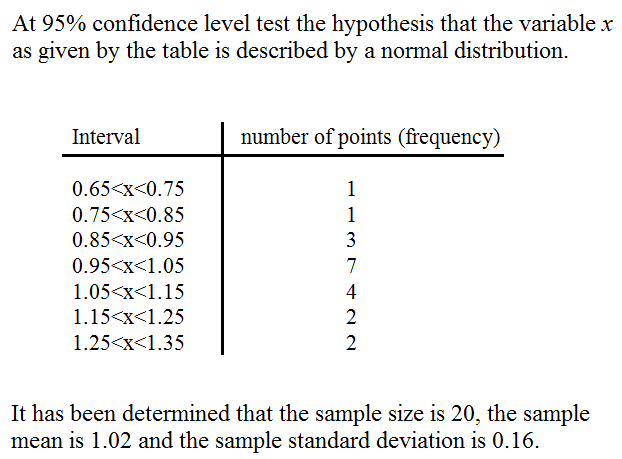# Chi-square Problem

Homework StatementThe attempt at a solution

I started by finding the expected frequencies corresponding to the intervals. I used the z-table for this. I got a chi-square value of 3.47.

The answer, however, should be 1.98.

Should I have used the z-table as mentioned earlier, or should I use the t-table? If so, how would I use the t-table?

Ray Vickson
Homework Helper
Dearly Missed
Homework StatementThe attempt at a solution

I started by finding the expected frequencies corresponding to the intervals. I used the z-table for this. I got a chi-square value of 3.47.

The answer, however, should be 1.98.

Should I have used the z-table as mentioned earlier, or should I use the t-table? If so, how would I use the t-table?

I got a chi-square value of 2.568.

You should NOT use the t-table, because the t-distribution applies to a completely different problem (estimating the mean)

RGV

I like Serena
Homework Helper
Yes, you should use the z-table, since that represents the normal distribution that you want to test against.

One of the preconditions for Pearson's chi-squared test is that the events must have a total probability of 1.
So we need to add 2 categories (x<0.65 and x>1.35) with observed frequency 0 for a total of 9 categories.
@RGV: Is it possible you missed that?

Using that I get a chi-square value of 3.17.
@Precursor: How did you get 3.47?

With 9-3=6 degrees of freedom that gives a p-value of 0.79.
Since a p-value of 0.05 is required, we can not reject the normal-distribution-hypothesis.

Ray Vickson
Homework Helper
Dearly Missed
Yes, you should use the z-table, since that represents the normal distribution that you want to test against.

One of the preconditions for Pearson's chi-squared test is that the events must have a total probability of 1.
So we need to add 2 categories (x<0.65 and x>1.35) with observed frequency 0 for a total of 9 categories.
@RGV: Is it possible you missed that?

Using that I get a chi-square value of 3.17.
@Precursor: How did you get 3.47?

With 9-3=6 degrees of freedom that gives a p-value of 0.79.
Since a p-value of 0.05 is required, we can not reject the normal-distribution-hypothesis.

Yes, the first time I did it I forget that; but I did it again (before you posted) with first category (-infinity,0.75) and final category (1.25,infinity) and got Chi^2 = 1.91255.

Your suggestion to take two new categories (< 0.65) and (>1.35) is NOT recommended: it is too arbitrary and is adding fictitious categories. For example, I could add a bunch of new categories, say (-infinity,0.05),(0.05,0.15),(0.15,0.25),(0.25,0.35),... , all with observed frequencies = 0 but non-zero theoretical frequencies. This would increase the value of Ch^2 and increase the number of degrees of freedom. The alternative, to expand the first one (0.65,0.75) into (-infinity,0.75) is more justifiable (and is essentially what the link you provide does near the bottom).

RGV

I like Serena
Homework Helper
Yes, the first time I did it I forget that; but I did it again (before you posted) with first category (-infinity,0.75) and final category (1.25,infinity) and got Chi^2 = 1.91255.

Your suggestion to take two new categories (< 0.65) and (>1.35) is NOT recommended: it is too arbitrary and is adding fictitious categories. For example, I could add a bunch of new categories, say (-infinity,0.05),(0.05,0.15),(0.15,0.25),(0.25,0.35),... , all with observed frequencies = 0 but non-zero theoretical frequencies. This would increase the value of Ch^2 and increase the number of degrees of freedom. The alternative, to expand the first one (0.65,0.75) into (-infinity,0.75) is more justifiable (and is essentially what the link you provide does near the bottom).

RGV

Nice!We're almost there (1.98).
I also draw the conclusion that calculating the chi-square test statistic gives you some freedom of choices that have quite some impact on the test statistic (although the resulting p-values are pretty close).
Adding a lot of fictitious categories does worsen the p-value significantly though.

I do wonder, aren't we throwing out some information here, since extending the category (0.65, 0.75) removes the information of the lower bound?